Courses

# Linear Algebra - MCQ Test

## 20 Questions MCQ Test GATE Electrical Engineering (EE) 2022 Mock Test Series | Linear Algebra - MCQ Test

Description
This mock test of Linear Algebra - MCQ Test for GATE helps you for every GATE entrance exam. This contains 20 Multiple Choice Questions for GATE Linear Algebra - MCQ Test (mcq) to study with solutions a complete question bank. The solved questions answers in this Linear Algebra - MCQ Test quiz give you a good mix of easy questions and tough questions. GATE students definitely take this Linear Algebra - MCQ Test exercise for a better result in the exam. You can find other Linear Algebra - MCQ Test extra questions, long questions & short questions for GATE on EduRev as well by searching above.
QUESTION: 1

### If A is a non–singular matrix and the eigen values of A are 2 , 3 , -3 then the eigen values of A-1 are

Solution:

If λ1 ,λ2 ,λ3 ....λare the eigen values of a non–singular matrix A, then A-1 has the eigen values  1/λ1 ,1/λ2 ,1/λ3 ....1/λn Thus eigen values of A-1are 1/2, 1/3, -1/3

QUESTION: 2

### If -1 , 2 , 3 are the eigen values of a square matrix A then the eigen values of A2 are

Solution:

If λ1 ,λ2 ,λ3 ....λare the eigen values of  a matrix A, then A2 has the eigen values  λ12 ,λ22 ,λ32 ....λn2 So, eigen values of Aare 1, 4, 9.

QUESTION: 3

### If 2 , - 4 are the eigen values of a non–singular matrix A and |A| = 4, then the eigen values of adjA are

Solution: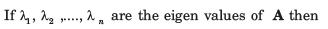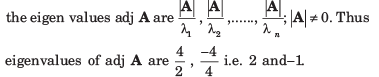QUESTION: 4

If 2 and 4 are the eigen values of A then the eigenvalues of AT are

Solution:

Since, the eigenvalues of A and Aare square so the eigenvalues of AT are 2 and 4.

QUESTION: 5

If 1 and 3 are the eigenvalues of a square matrix A then A3 is equal to

Solution:

Since 1 and 3 are the eigenvalues of A so the characteristic equation of A is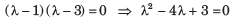Also, by Cayley–Hamilton theorem, every square matrix satisfies its own characteristic equation so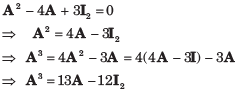QUESTION: 6

If A is a square matrix of order 3 and |A| = 2 then A (adj A) is equal to

Solution: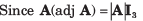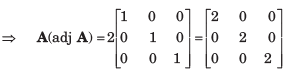QUESTION: 7

The sum of the eigenvalues of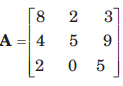is equal to

Solution:

Since the sum of the eigenvalues of an n–square matrix is equal to the trace of the matrix (i.e. sum of the diagonal elements) so, required sum = 8 + 5 + 5  = 18

QUESTION: 8

If 1, 2 and 5 are the eigen values of the matrix A then |A| is equal to

Solution:

Since the product of the eigenvalues is equal to the determinant of the matrix so |A| = 1 x 2 x 5 = 10

QUESTION: 9

If the product of matrices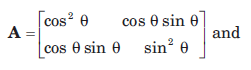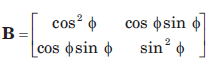is a null matrix, then θ and Ø differ by

Solution: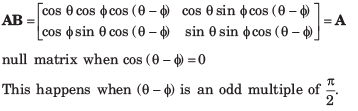QUESTION: 10

If A and B are two matrices such that A +  B and AB are both defined, then A and B are

Solution:

Since A + B is defined, A and B are matrices of the same type, say m x n. Also, AB is defined. So, the number of columns in A must be equal to the number of rows in B i.e. n = m. Hence, A and B are square matrices of the same order.

QUESTION: 11

If A is a 3-rowed square matrix such that |A| = 2, then |adj(adj A2)| is equal to

Solution:
QUESTION: 12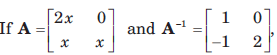then the value of x is

Solution: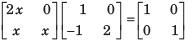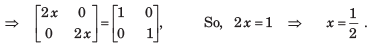QUESTION: 13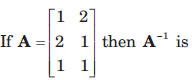Solution:

Inverse matrix is defined for square matrix only.

QUESTION: 14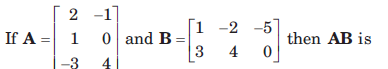Solution: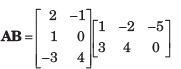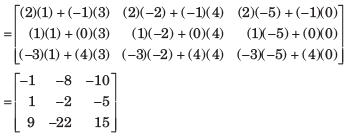QUESTION: 15

A set of linear equations is represented by the matrix equation Ax = b. The necessary condition for the existence of a solution for this system is

Solution:
QUESTION: 16

Select a suitable figure from the four alternatives that would complete the figure matrix.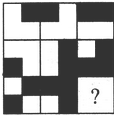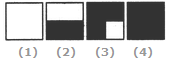Solution:

In each row (as well as each column), the third figure is a combination of all the elements of the first and the second figures

QUESTION: 17

For a skew symmetric even ordered matrix A of integers, which of the following will not hold true:

Solution:
QUESTION: 18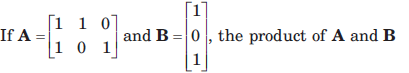Solution: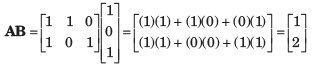QUESTION: 19

Matrix D is an orthogonal matrix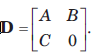The value of B is

Solution:

For orthogonal matrix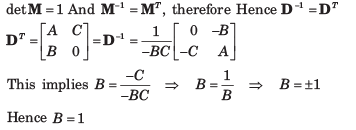QUESTION: 20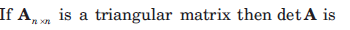Solution:

From linear algebra for Anxn triangular matrix . DetA =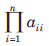The product of the diagonal entries of A